### Large Trapezoids for Pattern Block Set

Three large trapezoids for pattern block set.### Large Trapezoids for Pattern Block Set

Three large trapezoids for pattern block set.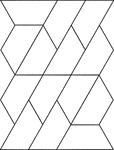### Small Trapezoids for Pattern Block Set

Fourteen small trapezoids for pattern block set.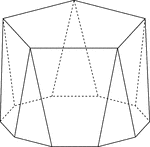### Polyhedron With Pentagon Bases

Illustration of a pentagonal polyhedron that is formed by having two parallel congruent pentagonal bases…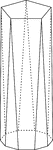### Polyhedron With Pentagon Bases

Illustration of a pentagonal polyhedron that is formed by having two parallel congruent pentagonal bases…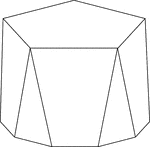### Polyhedron With Pentagon Bases

Illustration of a pentagonal polyhedron that is formed by having two parallel congruent pentagonal bases…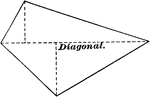### Trapezium

Illustration of a trapezium, a quadrilateral having no two sides parallel. The diagonal is labeled in…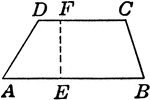### Trapezoid

Trapezoid with angles and height labeled.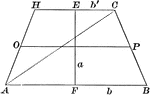### Area of a Trapezoid

Illustration of a trapezoid with altitude a and bases b and b' used to demonstrate that the area is…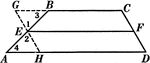### Line Bisecting Trapezoid Sides

Illustration used to prove the theorem "The line bisecting one of the non parallel sides of a trapezoid…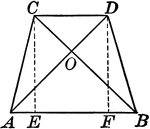### Isosceles Trapezoid With Equal Diagonals

Illustrations of an isosceles trapezoid with equal diagonals drawn.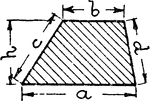### Trapezoid With Labels

An illustration of a trapezoid with 4 sides and height labeled.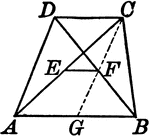### Trapezoid With Lines Joining the Midpoints of the Diagonals

Illustrations of a trapezoid with a line joining the midpoints of the diagonals.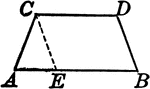### Trapezoid With Line Segment Parallel to One Side

Illustrations of a trapezoid with a line segment drawn parallel to one side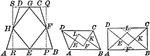### Trapezoids With Lines Joining Midpoints

Illustrations to show trapezoids with lines joining the midpoints of the sides of any quadrilateral,…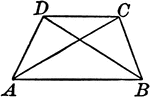### Trapezoid With Diagonals

Illustrations of a trapezoid with diagonals drawn.